Monday, December 31, 2018

New Years Eve Plans!

(Click on the comic if you can't see the full image.)(C)Copyright 2018, C. Burke.

Now I know why I like math puns so much. When people talk, it gets so wordy!

And there's a lot of standing around so that they get a chance to say this stuff.

And I deleted and rewrote a lot of dialogue.

Which is why this didn't actually appear on the last day before Christmas break!

I considered labeling this one "School Life", but I wanted to keep that for the students. And I've toyed with "After Hours" for the lives of the characters, but this is obviously school time. When else would Mike and Judy be around each other? Such is the life of a comic writer.

Oh, and Happy New Year! May all your plans be successful!

Come back often for more funny math and geeky comics.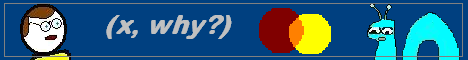Friday, December 28, 2018

(x, why?) Mini: Shapes Are Cool!

(Click on the comic if you can't see the full image.)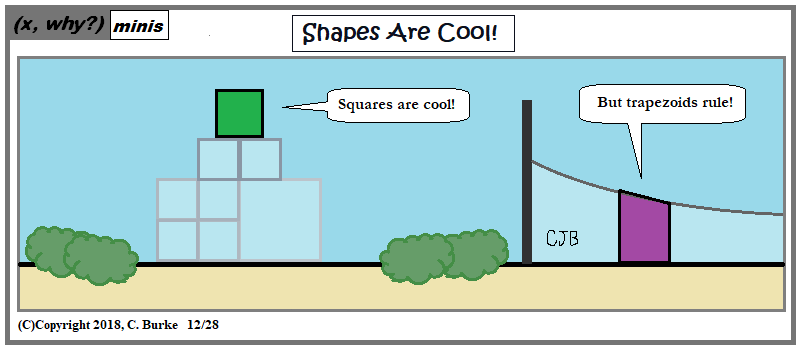(C)Copyright 2018, C. Burke.

Their shapes are compatible though trapezoids are less elegant. Trapezoids have no concept of elegance.

Come back often for more funny math and geeky comics.Thursday, December 27, 2018

Algebra 2 Problems of the Day

(not quite) Daily Algebra 2 questions and answers.

More Algebra 2 problems.

June 2017, Part IV

The Question in Part IV is worth up to 6 credits. Partial credit is possible.

37. A radioactive substance has a mass of 140 g at 3 p.m. and 100 g at 8 p.m. Write an equation in the form A = A0(1/2)(t/h) that models this situation, where h is the constant representing the number of hours in the half-life, A0 is the initial mass, and A is the mass t hours after 3 p.m.

Using this equation, solve for h, to the nearest ten thousandth.

Determine when the mass of the radioactive substance will be 40 g. Round your answer to the nearest tenth of an hour.

We are given A = 100, A0 = 140, and t = (8 - 3) = 5. We want h.
A = A0(1/2)(t/h)
100 = 140 (1/2)(5/h)
100/140 = (1/2)(5/h)
5 / 7 = (1/2)(5/h)
(log 5/7) / (log 1/2) = (5/h) log (1/2) / log (1/2)
(log 5/7) / (log 1/2) = 5 / h
(log 5/7) * h = (log 1/2) * 5
h = (log 1/2) / (log 5/7) * 5
h = 10.3002, to the nearest ten-thousandth.

Second part:
We are given A = 40, A0 = 140. We now have h = 10.3002. We want t.
A = A0(1/2)(t/h)
40 = 140 (1/2)(t/10.3002)
40 / 140 = (1/2)(t/10.3002)
2 / 7 = (1/2)(t/10.3002)
(log 2 / 7) / (log 1/2) = t / 10.3002
t = 10.3002 * (log 2/7) / (log 1/2)
t = 18.61... = 18.6 hours to the nearest tenth of an hour.

Logs were never my favorite subject in school, even if the rules are pretty straightforward. It's just one more inverse operation.

Next up: January 2017.

Comments and questions welcome.

More Algebra 2 problems.

Wednesday, December 26, 2018

Can Hardly Wait!

(Click on the comic if you can't see the full image.)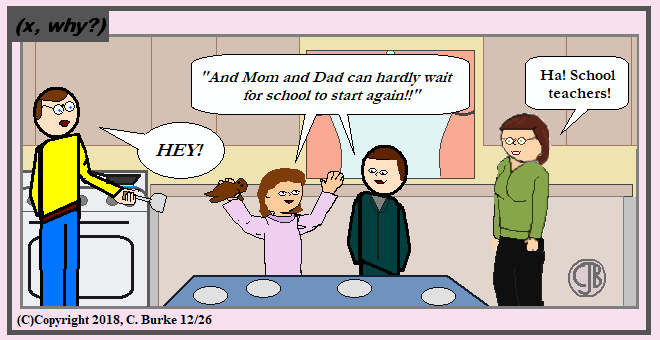(C)Copyright 2018, C. Burke.

Plot twist: Mom works outside of the house, too, but she took off this week to spend time with the kids!

Come back often for more funny math and geeky comics.Tuesday, December 25, 2018

Algebra 2 Problems of the Day

Daily Algebra 2 questions and answers.

More Algebra 2 problems.

June 2017, Part III

All Questions in Part III are worth up to 4 credits. Partial credit is possible.

35. Graph y = log2(x + 3) - 5 on the set of axes below. Use an appropriate scale to include both intercepts.

Describe the behavior of the given function as x approaches -3 and as x approaches positive infinity.

If you graph y = log2(x + 3) - 5, you will see that it is not defined for x %lt 3.
The y-intercept is at y = log2(0 + 3) - 5 = -3.42.
Solving 0 = log2(x + 3) - 5 gives you the x-intercept at 29.
You can use the calculator, or work it out:

0 = log2(x + 3) - 5
5 = log2(x + 3)
x + 3 = 25
x + 3 = 32
x = 29

The best scale to use for the x-axis is 3. Use a scale of 1 for the y-axis.
Check the image below. The table of values is provided for you. It wasn't necessary for the exam, but it would be a good idea to label the intercepts.

Second part: As x approaches -3, the function goes to negative infinity. As x approaches infinity, the function approaches infinity.

36. Charlie’s Automotive Dealership is considering implementing a new check-in procedure for customers who are bringing their vehicles for routine maintenance. The dealership will launch the procedure if 50% or more of the customers give the new procedure a favorable rating when compared to the current procedure. The dealership devises a simulation based on the minimal requirement that 50% of the customers prefer the new procedure. Each dot on the graph below represents the proportion of the customers who preferred the new check-in procedure, each of sample size 40, simulated 100 times.

Assume the set of data is approximately normal and the dealership wants to be 95% confident of its results. Determine an interval containing the plausible sample values for which the dealership will launch the new procedure. Round your answer to the nearest hundredth.

Forty customers are selected randomly to undergo the new check-in procedure and the proportion of customers who prefer the new procedure is 32.5%. The dealership decides not to implement the new check-in procedure based on the results of the study. Use statistical evidence to explain this decision.

The mean is given as 0.506, and one standard deviation is 0.078. To be 95% confident in the result requires two standard deviations, or 2 * 0.078 above or below the mean. So the interval would be:

0.506 - 2 * 0.078 < 0.506 < 0.506 + 2 * 0.078
0.506 - 0.156 < 0.506 < 0.506 + 2 * 0.156
0.354 < 0.506 < 0.656
0.35 < 0.506 < 0.66
Be sure to round to the nearest hundredth.

In the second part, since 32.5% is below 35.4%, the amount is outside of the 95% confidence interval.

Comments and questions welcome.

More Algebra 2 problems.

Monday, December 24, 2018

Christmas Once a Venn

(Click on the comic if you can't see the full image.)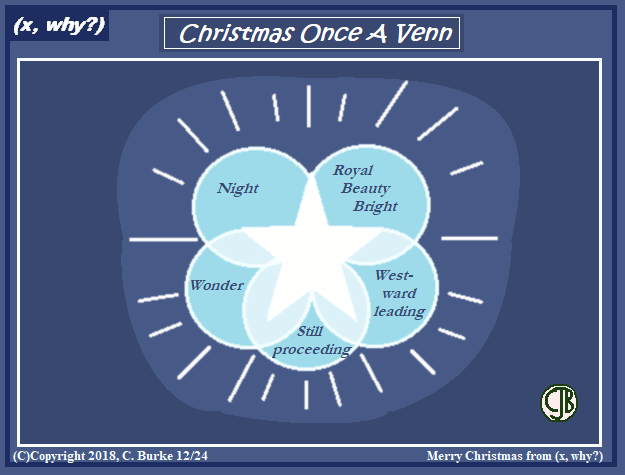(C)Copyright 2018, C. Burke.

Guide us to Thy perfect light!

Merry Christmas, from (x, why?)

Come back often for more funny math and geeky comics.Friday, December 21, 2018

No L

(Click on the comic if you can't see the full image.)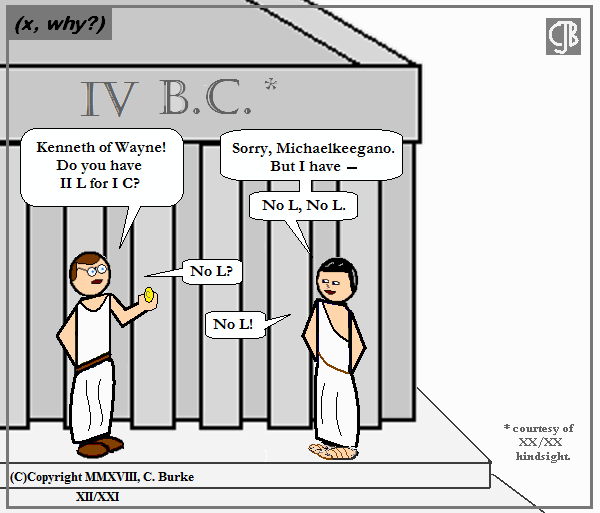(C)Copyright 2018, C. Burke.

I guess he needs to pay Caesar what's Caesar's. Everyone else is doing a "No L" comic, so why not, right?

Come back often for more funny math and geeky comics.Wednesday, December 19, 2018

Greetings

(Click on the comic if you can't see the full image.)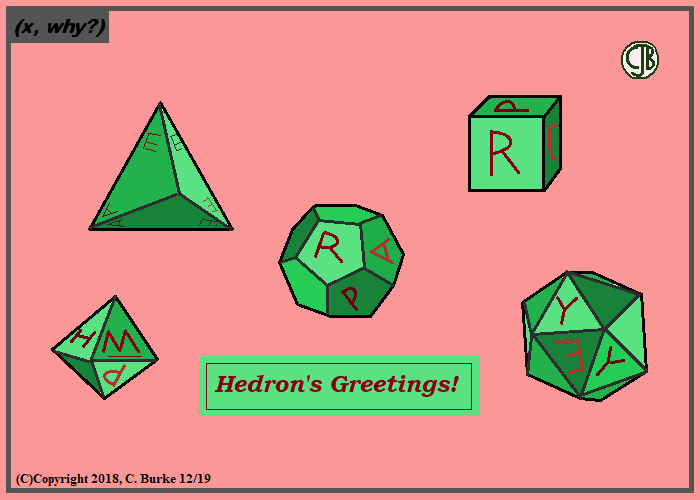(C)Copyright 2018, C. Burke.

I actually considered putting images of the 12 Days of Christmas on the dodecahedron. Then I got sane.

Seriously, after 5 Gold Rings, I wasn't getting anything onto those faces.

However, there are three words on the five dice.

Come back often for more funny math and geeky comics.Monday, December 17, 2018

Wassailing

(Click on the comic if you can't see the full image.)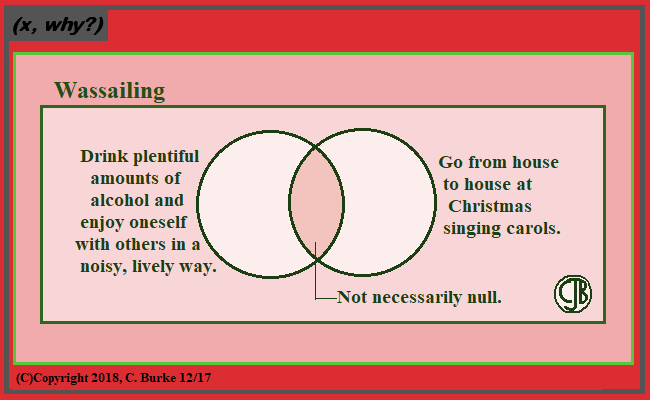(C)Copyright 2018, C. Burke.

Waaaaaaaaa-saaaaaaaaaaaaaaaail!

Of the two definitions, it's easy to see how one could lead to the other.

Come back often for more funny math and geeky comics.Friday, December 14, 2018

Dodec the Halls

(Click on the comic if you can't see the full image.)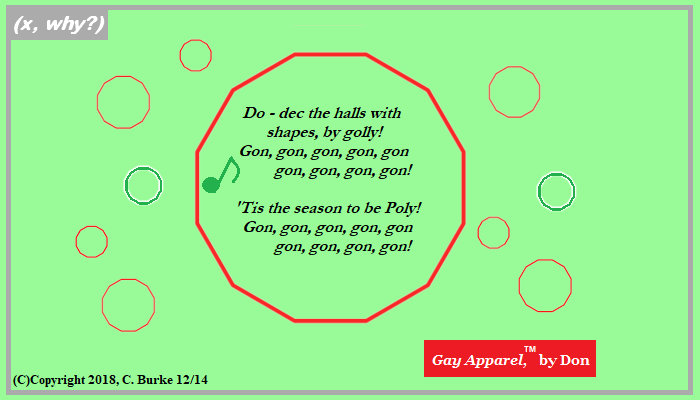(C)Copyright 2018, C. Burke.

Hang up your ''dodecorations''!

And I wish I could take credit for that, but someone actually made these!
I am not affiliated with these, but I've seen them on Twitter, and they're cute.
https://mathsgear.co.uk/products/dodecorations

Come back often for more funny math and geeky comics.Thursday, December 13, 2018

Skate Fall

(Click on the comic if you can't see the full image.)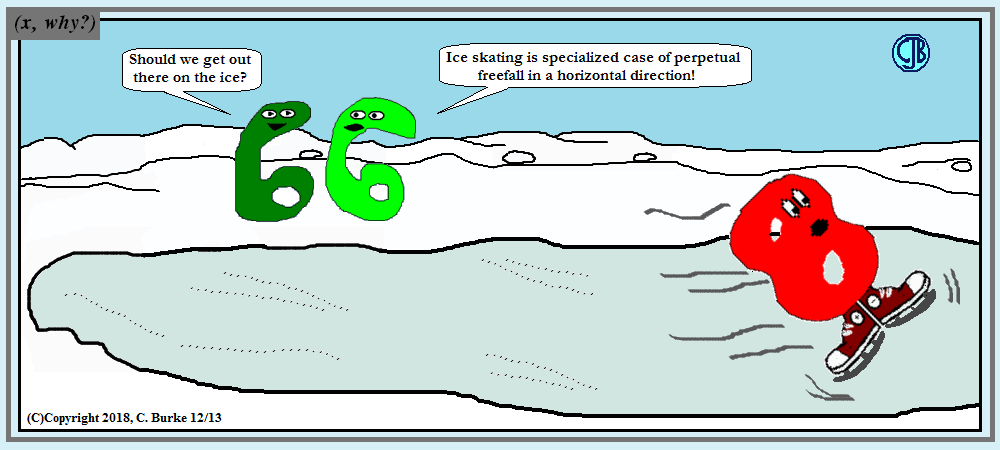(C)Copyright 2018, C. Burke.

Can a figure 8 make a figure 8? I'm lucky if both feet are headed in the same direction!

So this is another back burner comic. I think originally, it was to feature the Keegan children and I wasn't ready to draw them like that yet. One thing I do know is that I wrote down this comment in 2010! Probably after a school outing one Saturday to the rink at Bryant Park.

Come back often for more funny math and geeky comics.Sunday, December 09, 2018

Algebra 2 Problems of the Day

Daily Algebra 2 questions and answers.

More Algebra 2 problems.

June 2017, Part III

All Questions in Part III are worth up to 4 credits. Partial credit is possible.

33. Solve the following system of equations algebraically for all values of x, y, and z:

x + y + z = 1
2x + 4y + 6z = 2
-x + 3y - 5z = 11

Any system of three equations with three variables can be solved by eliminating one of the variables and then solving the remaining system of equations with two variables.

I was away from the PC for a while the other day, so I worked the solution out long hand, two different ways. First by using elimination, then by using substitution, to remove the x. After that, the work would be the same.

Note that the 2x + 2y + 2z = 2 came from multiplying the first equation by 2.
At this point, by either method, you should be left with the two equations:

2y + 4z = 0 and
4y - 4z = 12
Add these together, and the z term is eliminated, allowing you to solve for y.
Once you have y, you can find z.
Once you have y and z, you can find x.

34. Jim is looking to buy a vacation home for \$172,600 near his favorite southern beach. The formula to compute a mortgage payment, M, is M = P • ( r (1+r)N) / ( (1 + r)N - 1) where P is the principal amount of the loan, r is the monthly interest rate, and N is the number of monthly payments. Jim’s bank offers a monthly interest rate of 0.305% for a 15-year mortgage.

With no down payment, determine Jim’s mortgage payment, rounded to the nearest dollar.

Algebraically determine and state the down payment, rounded to the nearest dollar, that Jim needs to make in order for his mortgage payment to be \$1100.

For the first part of the question, use the given formula with the following substitutions:
P = 172,600, r = 0.00305, N = 12 * 15 = 180 payments
M = P • ( r (1+r)N) / ( (1 + r)N - 1)
M = 172600 • ( 0.00305 (1+0.00305)180) / ( (1 + 0.00305)180 - 1)
M = 1247.4933... = 1247 to the nearest dollar. (Round 49 cents down.)

The down payment reduces the Principal that needs to be borrowed. To answer the second part, we need to solve for P when M = 1100. Once we know that, we need to subtract 172600 - P to find the amount of the down payment.
M = P • ( r (1+r)N) / ( (1 + r)N - 1)
1100 = P • ( 0.00305 (1+0.00305)180) / ( (1 + 0.00305)180 - 1)
P = 1100 / • ( 0.00305 (1+0.00305)180) / ( (1 + 0.00305)180 - 1)
P = 152193.19...
The down payment is 172600 - 152193.19 = 20406.81 = 20407.

Comments and questions welcome.

More Algebra 2 problems.

Friday, December 07, 2018

(x, why?) Mini: Revolution

(Click on the comic if you can't see the full image.)(C)Copyright 2018, C. Burke.

Why, yes, I have been covering science classes again. Why do you ask?

Come back often for more funny math and geeky comics.Thursday, December 06, 2018

Algebra 2 Problems of the Day

Daily Algebra 2 questions and answers.

More Algebra 2 problems.

June 2017, Part II

All Questions in Part II are worth up tp 2 credits. Partial credit is possible.

31. Write (x)(1/3) * (x)(1/2) as a single term with a rational exponent.

First of all, please look at the image. This is how the question was asked. I typed it the best way that I could for this medium.
That said, you're first step is to rewrite the equation as:

(x)(1/3) * (x)(1/2)

Now use the rules of exponents:
(x)(1/3) * (x)(1/2) = (x)(5/6)

Leave your answer in this form with the rational (fractional) exponent. Do NOT rewrite it with a radical symbol.

Note: (1/3) + (1/2) = (2/6) + (3/6) = (5/6)

32. Data collected about jogging from students with two older siblings in the table below.

 Neither Sibling Jogs One Sibling Jogs Both Sibling Jog Student Does Not Jog 1168 1823 1380 Student Jogs 188 416 400

Using these data, determine whether a student with two older siblings is more likely to jog if one sibling jogs or if both siblings job. Justify your answer.

Sum up the second and the third columns. The number of students with one sibling who jogs is 1823 + 416 = 2239. The number of students with two siblings who jog is 1380 + 400 = 1780.

The probability that a student jogs if one sibling jogs is
P(student | one sibling) = 416/2239 = .186

The probability that a student jogs if both siblings jog is
P(student | both sibling) = 400/1780 = .225

It is more likely that a student with two older siblings will jog if both of their older siblings jog.

Comments and questions welcome.

More Algebra 2 problems.

Tuesday, December 04, 2018

Algebra 2 Problems of the Day

Daily Algebra 2 questions and answers.

More Algebra 2 problems.

June 2017, Part II

All Questions in Part II are worth up tp 2 credits. Partial credit is possible.

29. Graph y = 400(.85)2x - 6 on the set of axes below. (image omitted, see below)

See the graph below.
The x-axis has a scale of 0.5, but using integer values for x is sufficient for full credit. The y-axis has a scale of 20.
I included a table of values for reference. It isn't needed, but it's not a bad idea to include it, or to label the points.
This was a very straightforward probably, using the graphing calculator. A little time-consuming, but simple.

30.Solve algebraically for all values of x:

sqrt(x - 4) + x = 6

Follow along on the image below. Subtract x from both sides of the equation to isolate the radical. Next, square both sides of the equation. Subtract (x - 4) from both sides to set the quadratic equation equal to 0. Factor the trinomial.

Comments and questions welcome.

More Algebra 2 problems.

Monday, December 03, 2018

Elemeno P

(Click on the comic if you can't see the full image.)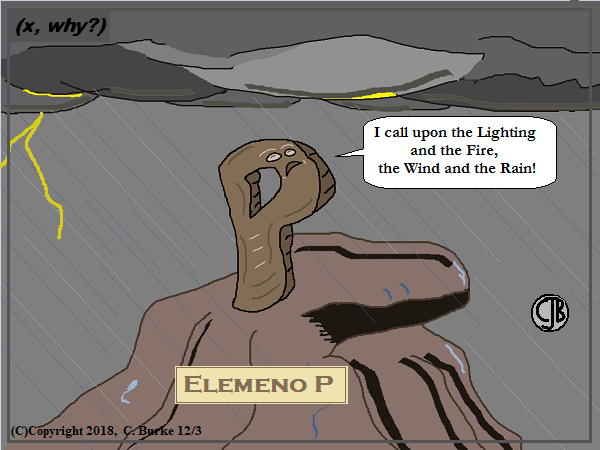(C)Copyright 2018, C. Burke.

''Elemental P'' might've been a rockier pun.

This is the dude from the Alphabet Song.

Come back often for more funny math and geeky comics.Saturday, December 01, 2018

Algebra 2 Problems of the Day

Daily Algebra 2 questions and answers.

More Algebra 2 problems.

June 2017, Part II

All Questions in Part II are worth up tp 2 credits. Partial credit is possible.

27.Over the set of integers, factor the expression 4x3 - x2 + 16x - 4 completely.

Group the terms into the pairs. You will notice that in the first two terms, you can factor out x2, as follows: x2(4x - 1).
In the last two terms, you can factor out a 4, as follows: (4)(4x - 1).
This gives you x2(4x - 1) + (4)(4x - 1).
Factor out the (4x - 1) from the two terms, and you get (x2 + 4)(4x - 1).
(Distributive Property in reverse.)
You can check your answer by multiplying (FOIL, box method) and getting your original polynomial back.

28. The graph below represents the height above the ground, h, in inches, of a point on a triathlete's bike wheel during a training ride in terms of time, t, in seconds.

Identify the period of the graph and describe what the period represents in this context.

First of all, you don't not need to know what a "triathlete" is, other than that it's someone who is riding a bike. Okay? Don't freak out over the word if you've never seen it before.
The period of the graph is 2/3, which you can see because the graph starts at a height of 26 and returns to 26 after 2/3 seconds.
The period represents the time it takes for a point at the top of the wheel to make one complete revolution and return back to the top of the wheel.

Comments and questions welcome.

More Algebra 2 problems.

Friday, November 30, 2018

Random Sketch

(Click on the comic if you can't see the full image.)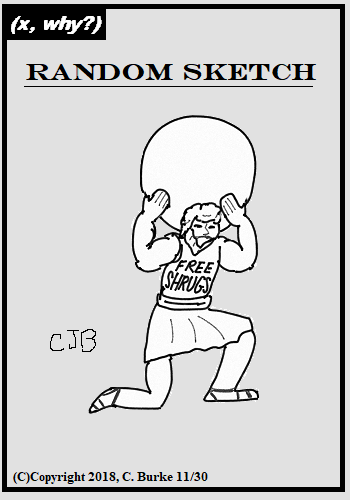(C)Copyright 2018, C. Burke.

Emphasis on the 'RANDOM' as it was just one of those things that occurs to me...

Come back often for more funny math and geeky comics.Wednesday, November 28, 2018

Colors Like My Screen

(Click on the comic if you can't see the full image.)(C)Copyright 2018, C. Burke.

Sometimes I'm like a dog with a pun, er, bone, and I don't want to let it go.

Come back often for more funny math and geeky comics.Tuesday, November 27, 2018

Algebra 2 Problems of the Day

Daily Algebra 2 questions and answers.

More Algebra 2 problems.

June 2017, Part II

All Questions in Part II are worth up tp 2 credits. Partial credit is possible.

25. Given r(x) = x3 - 4x2 + 4x - 6, find the value of r(2).

What does your answer tell you about x - 2 as a factor of r(x)? Explain.

r(2) = (2)3 - 4(2)x2 + 4(2) - 6
r(2) = 8 - 4(4) + 8 - 6 = 8 - 16 + 8 - 6 = -6
Therefore, x - 2 is not a factor of r(x) because r(2) =/= 0. If you divide r(x) / (x - 2) there will be a remainder.

26. The weight of a bag of pears at the local market averages 8 pounds with a standard deviation of 0.5 pound. The weights of all the bags of pears at the market closely follow a normal distribution. Determine what percentage of bags, to the nearest integer, weighed less than 8.25 pounds.

Use your calculator to find a normal distribution. "less than 8.25" means that the endpoints are 0 and 8.25. The mean is 8 and the standard deviation is 0.5.
On your paper, write normalcdf(0,8.25,8,.50) to show work to get full credit. Then enter this into your calculator.
You will get an answer of .6915, which is 69% to the nearest integer.

Comments and questions welcome.

More Algebra 2 problems.

Monday, November 26, 2018

How To Tell the Contradiction

(Click on the comic if you can't see the full image.)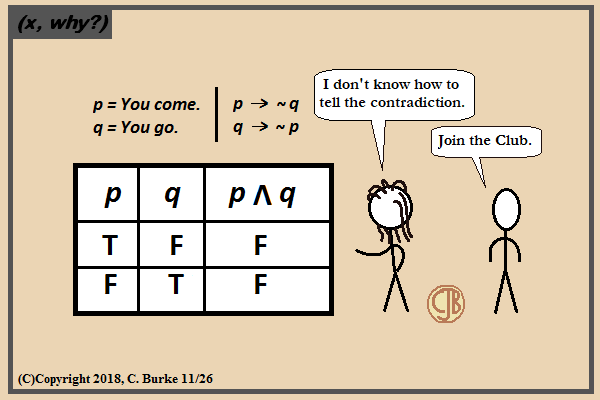(C)Copyright 2018, C. Burke.

Loving would easy if your numbers were like my dreams: fourteen oh three, fourteen oh thre-ee-ee!!

The original truth table for this was going to be way too complicated for this joke.

Oh, and if you're reading this on the blog, this is comic #1403, which just worked out that way. I pretty much just needed a three at the end.

Come back often for more funny math and geeky comics.Sunday, November 25, 2018

Algebra 2 Problems of the Day

Daily Algebra 2 questions and answers.

More Algebra 2 problems.

June 2017, Part I

All Questions in Part I are worth 2 credits. No work need be shown. No partial credit.

22. Mallory wants to buy a new window air conditioning unit. The cost for the unit is \$329.99. If she plans to run the unit three months out of the year for an annual operating cost of \$108.78, which function models the cost per year over the lifetime of the unit, C(n), in terms of the number of years, n, that she owns the air conditioner?
(1) C(n) = 329.99 + 108.78n
(2) C(n) = 329.99 + 326.34n
(3) C(n) = (329.99 + 108.78n) / n
(4) C(n) = (329.99 + 326.34n) / n

Answer: (3) C(n) = (329.99 + 108.78n) / n
Choice (1) is the total cost to operate the unit over its lifetime. To find the yearly cost, divide that amount by the number of years, n.
Alternatively, if it costs \$108.78 to operate per year (and then the cost is 108.78n to operate for n years). The cost of the unit is paid once (initial value) and divided by the number of years the unit is owned to get a "per year" cost, which is 329.99/n.
So the yearly cost is: 329.99/n + 108.78, which is equivalent to (329.99 + 108.78n) / n.

23. The expression (-3x2 - 5x + 2) / (x3 + 2x2)

can be rewritten as

Answer: (4) -3x-1 + x-2
As shown in the image below. Factor both the numerator and the denominator.
The numerator is (-1)(3x2 + 5x - 2), which factors into (-1)(3x - 1)(x + 2).
The denominator is (x2)(x + 2).
Eliminate the common factor of x + 2.
At this point, choice (2) looks so close to being correct, but it has a numerator of -3x - 1, instead of -3x + 1.
Use the division rule and reduce the exponents in the numerator by 2, remembering that the last term is actually 1x0.
This gives us choice (4).

24.Jasmine decides to put \$100 in a savings account each month. The account pays 3% annual interest, compounded monthly. How much money, S, will Jasmine have after one year?

Answer: (2) S = (100 - 100(1.0025)12)/(1 - 1.0025)
When compounding interest, if \$100 were deposited initially, with no additional deposits, then choice (3) would have been correct. The interest rat of 3%, or 0.03, is spread out over 12 months. In other words, 0.03/12 = 0.0025 is the monthly interest, raised to the power of 12 for the entire year. You can eliminate choices (1) and (4), both of which use 1.03.
Because additional deposits are being made monthly, choice (3) is incorrect. The more complicated formula shown in choice (2) comes from the Geometric Sequence formula in the reference table at the back of the booklet:

Sn = (a1 - a1rn) / (1 - r), where r =/= 1.
In this case, a1 = 100, and r = 1.0025.

Comments and questions welcome.

More Algebra 2 problems.

Thursday, November 22, 2018

Happy Thanksgiving 2018!

(Click on the comic if you can't see the full image.)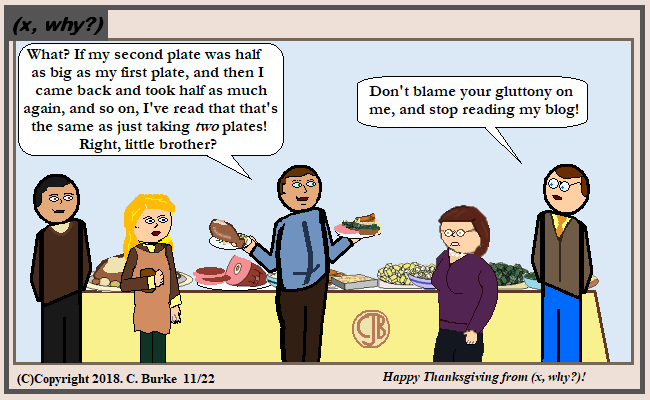(C)Copyright 2018, C. Burke.

Continually taking half as much as the last time is not likely a reasonable assumption. Making an infinite number of trips for more food, on the other hand ...

Happy Thanksgiving, from (x, why?)!

Come back often for more funny math and geeky comics.Tuesday, November 20, 2018

Romaine Numerals

(Click on the comic if you can't see the full image.)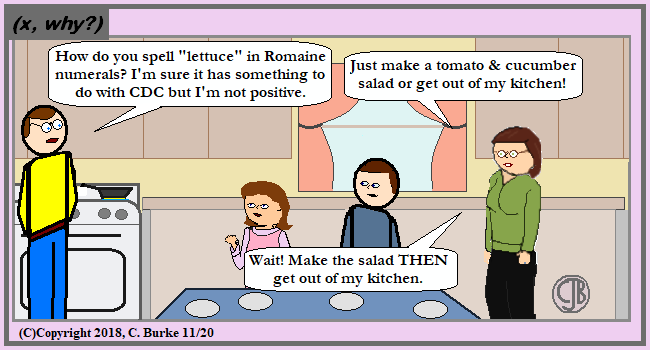(C)Copyright 2018, C. Burke.

Actually, I guess 'CDC' would just be 'D'.

Now "lettuce" dispense with all the other puns, shall we?

Come back often for more funny math and geeky comics.Friday, November 16, 2018

Units of Pizza

(Click on the comic if you can't see the full image.)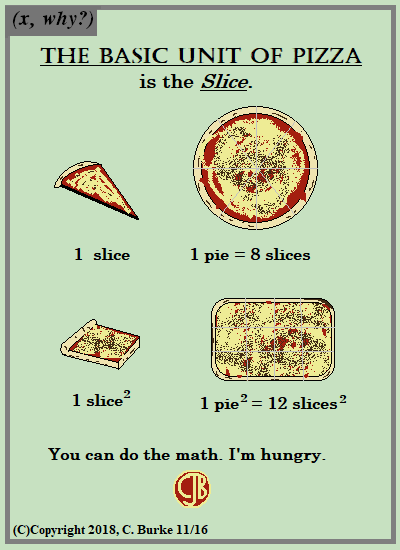(C)Copyright 2018, C. Burke.

Rankings of Best Pizza: Brooklyn; rest of NYC; every place else, does it matter?

Rankings of Best Pizza chains:

Come back often for more funny math and geeky comics.Wednesday, November 14, 2018

R. I. P. Stan Lee

(Click on the comic if you can't see the full image.)(C)Copyright 2018, C. Burke.

Excelsior!

I've tried to get away from doing obit comics, but this was one passing that needed to be marked. I knew who Stan Lee was at an early age, even if I didn't read Marvel comics very much. They were a little difficult to get into if you didn't have a good entry point. I prefered the cartoons -- by which I mean "Spider-man! Spider-man! Does what every a spider can!" and "When Captain America throws his mighty shield!"

Yes, I'm old. But you knew that.

It was amazing how quickly the meme pile-up was in this case though.

Come back often for more funny math and geeky comics.Tuesday, November 13, 2018

Algebra 2 Problems of the Day

Daily Algebra 2 questions and answers.

More Algebra 2 problems.

June 2017, Part I

All Questions in Part I are worth 2 credits. No work need be shown. No partial credit.

19. To solve,

Ren multiplied both sides by the least common denominator. Which statement is true?
(1) 2 is an extraneous solution
(2) 7/2 is an extraneous solution
(3) 0 and 2 are extraneous solutions
(4) This equation does not contain any extraneous solutions.

Answer: (1) 2 is an extraneous solution.
The equation is undefined for 0 and 2 because of the terms in the denominator. When both sides are multiplied by x2 - 2x, that restriction is lifted. If either 0 or 2 are solutions to the equation, then they are extraneous.
Look at the steps below:

At this point, you can check both 0 and 2 to see if they are solutions to the equation.
2(0)2 - 11(0 - 2) = 0 - 11(-2) = 22, which is not equal to 8. Zero is not an extraneous solution.
2(2)2 - 11(2 - 2) = 2(4) - 11(0) = 8 - 0 = 8. Two is an extraneous solution.
As for 7/2, if you finish working out the problem, you will see that it is a solution to the equation, but it is not an extraneous solution.

20. Given f(9) = -2, which function can be used to generate the sequence -8, -7.25, -6.5, -5.75,...?
(1) f(n) = -8 + 0.75n
(2) f(n) = -8 - 0.75(n - 1)
(3) f(n) = -8.75 + 0.75n
(4) f(n) = -0.75 = 8(n - 1)

Answer: (3) f(n) = -8.75 + 0.75n
The rate of change is +0.75, which eliminates (2) and (4).
Choice (1) is incorrect because it has an incorrect starting value. If you substitute n = 1, you get -7.25 instead of 8.
Choice (2) would have been correct if there had been addition instead of subtraction.
Choice (3) is the correct answer because adding 0.75 to -8.75 gives you the initial term of -8.

21. The function

represents a damped sound wave function. What is the average rate of change for this function on the interval [-7,7], to the nearest hundredth?
(1) -3.66
(2) -0.30
(3) -0.26
(4) 3.36